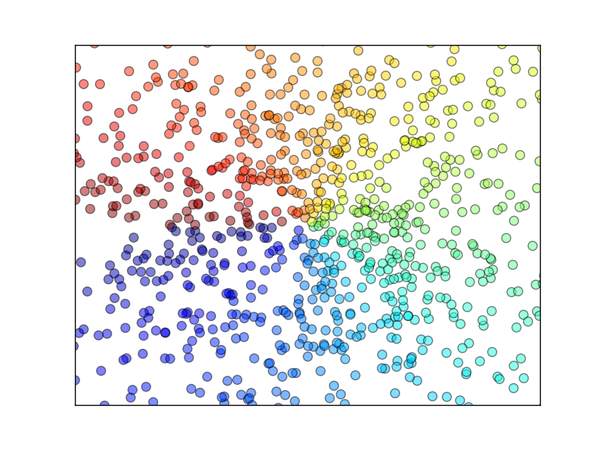# Matplotlib scatter 散点图

639℃## 散点图

``````import matplotlib.pyplot as plt
import numpy as np

n = 1024    # data size
X = np.random.normal(0, 1, n) # 每一个点的X值
Y = np.random.normal(0, 1, n) # 每一个点的Y值
T = np.arctan2(Y,X) # for color value````````````plt.scatter(X, Y, s=75, c=T, alpha=.5)

plt.xlim(-1.5, 1.5)
plt.xticks(())  # ignore xticks
plt.ylim(-1.5, 1.5)
plt.yticks(())  # ignore yticks

plt.show()``````